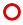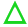## Not all lifts are born equal

Let’s return to the same example as for the previous section about “CRM tools”. Let’s assume that you want to do a direct-mail marketing campaign for one of your product. You must find all the customers that are susceptible to buy your product and send them a brochure or leaflet (this is a classical “propensity to buy” setting but the same reasoning applies to any other settings: cross-selling, upselling, probability of default, etc.).

• The individuals are represented either with aor a.
The targets (the people that have bought your product) are represented with thesign.
• The blue line represents a good predictive model (model 1). Basically the model says “You are a target if your age is below 42” (i.e. “you will buy the product if your age is below 42”).

Please Note that this first model is making some errors (in total there are 4 errors): i.e. there are threethat are classified asand onethat is classified as a.

These errors are pointed in the illustration below:Based on the population illustrated above, we could have created another second model that is is making less errors on the “training dataset”. The population (or dataset) that is used to create a predictive model is named the “training dataset”.

Let’s now have a look at a second model (model 2) illustrated below:This last model looks better because it’s making absolutely no error on the “learning/creation dataset” (the lift of the learning dataset will be 100% accurate).

Let’s now use these two models on an unknown, new dataset (a new population), classically named the “TEST dataset” (since it’s a new, unseen population the position of theand theis slightly different):

We can see that, when we apply the two models on the “test dataset”, the first model is really better: it makes no errors. In opposition, the second model makes 9 errors.

The phenomenon illustrated above is the following: When we are constructing a model that makes no mistakes on the “training dataset”, we obtain a model that does NOT perform well on unseen data. The “generalization ability” of our model is poor. The predictive model is using some information there were in reality noises. This phenomenon is named “Over-fitting”: we are using some information out of the “learning dataset” but we shouldn’t. We are learning the noise contained inside the “learning dataset”.

In the example above, we will say that the “Model 2” “overfits” the data and, thus, it can’t be used. “Model 1” does not overfit the data and can be used.

### Some important facts

A model that “overfits” the data can have an accuracy of 100% when applied on the learning set.

When comparing the accuracy of 2 different predictive models to know which predictive model is the best one (the one with the highest accuracy & the highest ROI), you must compare ONLY the lift curves computed on the TEST dataset and if possible, use always the same TEST dataset.

In some extreme (but common) “over-fitting” situation, the predictive model can be completely wrong because the predictions are computed based on completely erroneous, un-meaningful criteria’s about your individuals. When you use such predictive model on new, unseen data (i.e. on new individuals) (i.e. on a “Test dataset”), it will give you many “wrong answer” (the predictive model is saying that some particular individuals will buy your product but in reality, they won’t).

You must avoid using “over-fitted” models. You can recognize them easily: they have a very good accuracy on the “learning/creation dataset” and have a very inferior accuracy on the “Test Dataset”. For example, in the chart below, there are 5 different predictive models. Each one of the 5 predictive models has been applied on its own “learning dataset” (curves in Red) and also on a separate “test dataset” (curves in Blue) , to create in total 10 (=5×2) different lifts. These 10 lifts are illustrated here:The 5 lifts in red are “the 5 predictive models” applied on their respsective creation set.
The 5 lifts in blue are “the 5 predictive models” applied on a separate test set.

The accuracy of these 5 models is completely different between the creation dataset and the TEST dataset. Thus, these 5 models are all “overfitting the data” and they can’t be used.

### Summary

To compare the accuracy of 2 predictive models: look only at the lift curves computed on the TEST dataset.